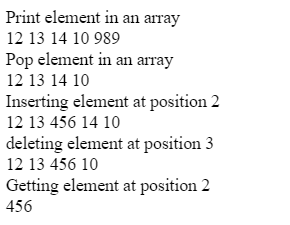# Implementation of Array class in JavaScript

This article implementing Arrays using JavaScript. An Array is a simple data Structure, in which elements are stored in contiguous memory locations. Implementation of arrays performs various operations like push (adding element), pop (deleting element) element at the end of the array, getting the element from particular index, inserting and deleting element from particular index.

Array class in JavaScript:

 `// User defined class Array ` `class Array { ` ` `  `    ``// Create constructor ` `    ``constructor() {   ` `     `  `        ``// It store the length of array. ` `        ``this``.length = 0;  ` `         `  `        ``// Object to store elements. ` `        ``this``.data = {};  ` `    ``} ` `} `

In the above example, create a class Array which contains two properties i.e. length and data, where length will store the length of an array and data is an object which is used to store elements.

Function in Array: There are many functions in array which are listed below:

• Push()
• Pop()
• insertAt()
• deleteAt()
• getElementAtIndex()

Push(element): This function is used to push an element at the end of the array.

 `push(element) { ` `    ``this``.data[``this``.length] = element; ` `    ``this``.length++; ` `    ``return` `this``.data; ` `} `

Pop(): It is used to delete an element at the end of the array.

 `pop() { ` `    ``let item = ``this``.data[``this``.length-1]; ` `    ``delete` `this``.data[``this``.length-1]; ` `    ``this``.length--; ` `    ``return` `this``.data; ` `} `

In the above example, item variable will store the last element from data object and perform deletion of last element and then, it will decrease the length of by 1 and return the object.

insertAt(): This function is used to insert an element at given index.

 `insertAt(item, index) { ` `    ``for``(let i=``this``.length;i>=index;i--) { ` `      ``this``.data[i]=``this``.data[i-1]; ` `    ``} ` `    ``this``.data[index]=item; ` `    ``this``.length++;  ` `    ``return` `this``.data; ` `} `

This function accepts two parameters item and index. Index number denoting the place where data to be inserted and item is the value which is to be inserted at index.

deleteAt(index): This function is used to remove an element at given index or property in a data object.

 `deleteAt(index) { ` `    ``for``(let i = index; i < ``this``.length - 1; i++) { ` `      ``this``.data[i] = ``this``.data[i+1]; ` `    ``} ` `    ``delete` `this``.data[``this``.length-1]; ` `    ``this``.length--; ` `    ``return` `this``.data; ` `} `

In above function, use loop to reach at index till the end, and copy the next element at index and at the end of loop two copies of last element exist, delete last element through delete operator.

getElementAtIndex(index): It returns the element at given index.

 `getElementAtIndex(index) { ` `    ``return` `this``.data[index]; ` `} `

Example: This function describes the implementation of array class and its various operations.

 ` ` ` ` ` `  ` ` `    `` ` `        ``Implementation of array ` `    `` ` ` ` ` `  ` ` `    `` ` ` ` `                     `

Output:My Personal Notes arrow_drop_upCheck out this Author's contributed articles.

If you like GeeksforGeeks and would like to contribute, you can also write an article using contribute.geeksforgeeks.org or mail your article to contribute@geeksforgeeks.org. See your article appearing on the GeeksforGeeks main page and help other Geeks.

Please Improve this article if you find anything incorrect by clicking on the "Improve Article" button below.